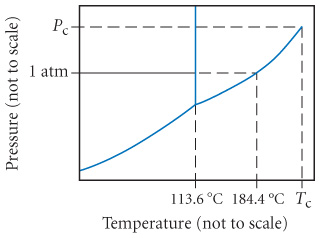# Problem: Consider the phase diagram for iodine shown below and answer each of the following questions.What is the melting point for iodine at 1 atm?

⚠️Our tutors found the solution shown to be helpful for the problem you're searching for. We don't have the exact solution yet.

###### Problem Details

Consider the phase diagram for iodine shown below and answer each of the following questions.What is the melting point for iodine at 1 atm?